Video Transcript of:

A Laplacian for Nonmanifold Triangle Meshes - SGP 2020

(Authors: Nicholas Sharp and Keenan Crane)

Transcript by http://rodolphe-vaillant.fr

Related source code:

https://github.com/nmwsharp/nonmanifold-laplacian

https://geometry-central.net/

Hi there everyone I'm Nick sharp and as part of SGP 2020 I'm excited to present our work on a laplacian for non manifold triangle meshes and point clouds.

So today we're interested in the discrete laplacian which discretizes the Laplace-Beltrami operator Delta. This takes the form of V by V matrix where V is the number of vertices in a triangle mesh: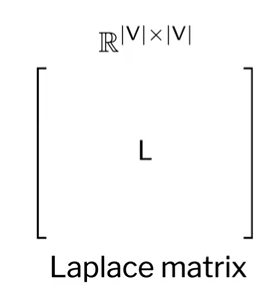and gets applied to scalar values to find at vertices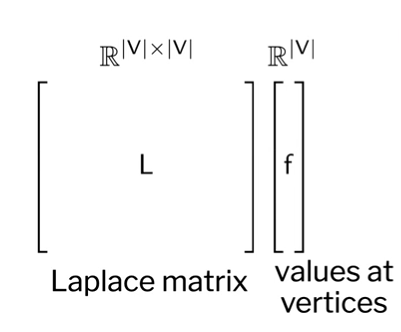the discrete laplacian is an extremely common ingredient in geometry processing algorithms for things like:

• shape deformation
• parameterization
• shape descriptors and so on and so forthin this work we'll develop a new algorithm which takes as input any triangle mesh which might be non manifold non-orientable or have boundary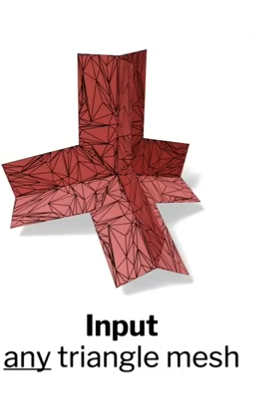and outputs a high quality laplace matrix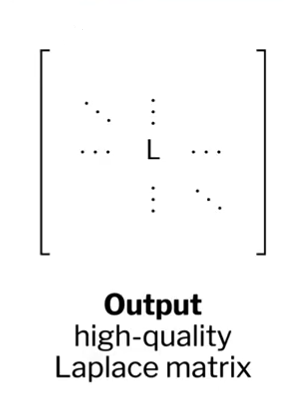we're really excited about this because it's one of the rare cases where we can just wave our hands and make a whole bunch of useful algorithms work better.

The Laplacian matrix we generate is a plain old V by V matrix, and it can simply be dropped in as a replacement for the ordinary cotangent laplacian improving the robustness and performance of many classical algorithms.

A little more precisely our contributions are to build a good Laplacian for any triangle mesh

• it might be non-orientable and on manifold or had a boundary
• and this Laplacian will have non-negative edge weights even in the presence of boundary
• and generally be more accurate than cotangent laplacian
• we also described a simple intrinsic mollification scheme which handles degenerate elements

We also show how the same construction can be used to get a point cloud laplacian with similar properties.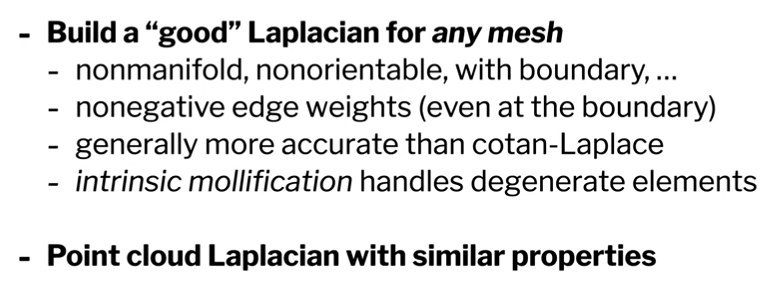A little more formally what we're doing here is extending the application of intrinsic Delaunay triangulations to non manifold geometry for the first time. The key idea that makes our method work, is that we sacrifice vertex manifoldness for edge manifoldness by building what we call the tufted cover: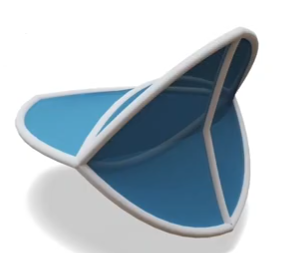But before you get too deep into the details a little context. This advancement is a piece of a larger effort in robust geometry processing. We've observed that formulating algorithms on ideal meshes often leads to systems , algorithms or methods which don't work so well on real data.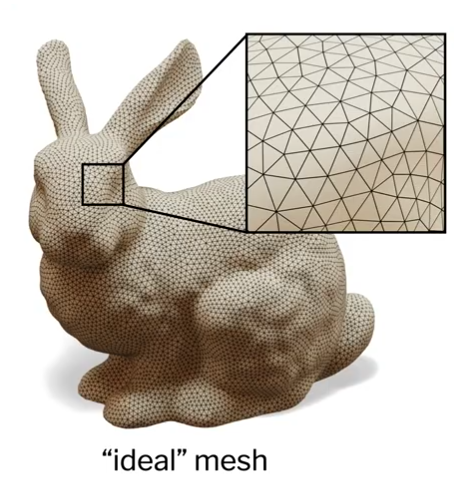In particular you'll notice that our ideal mesh here has

• regular sampling of vertices
• has equilateral triangles

whereas CAD meshes or meshes from a 3d scanning system: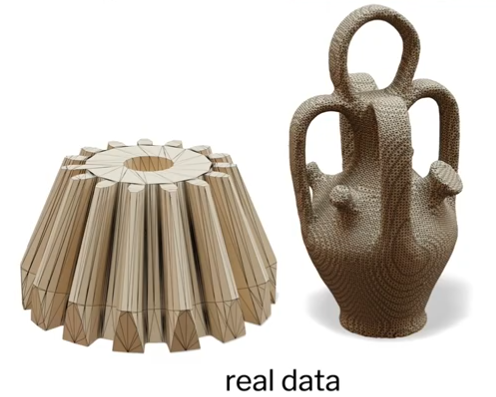might have

• an irregular sample in your vertices
• and lots of skinny triangles

Algorithms formulated on this ideal mesh might crash and burn when you try to run them on real data. So we're searching for techniques which yield reasonable results across a very very broad range of possible inputs and don't impose these kinds of restrictions.

There are a lot of things that can potentially be wrong with a mesh. Most simply your mesh might encode the wrong geometry. So it could have holes in it or spurious features but today we're actually not going to talk about this too much: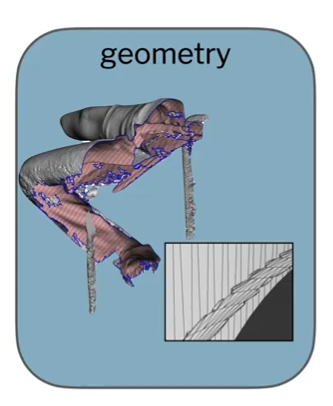Moving forward there's always an assumption that the mesh you have somehow corresponds to a surface you care about, however, you still might be worried about problems with floating point for instance: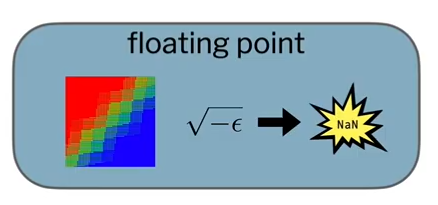Where you care not so much just about inaccurate solutions but also about structural problems, like getting out all NaNs after writing your algorithm.

Even if you could compute directly with real numbers instead of in floating-point you'd still have to be concerned about low quality elements like skinny triangles with very obtuse or very acute angles: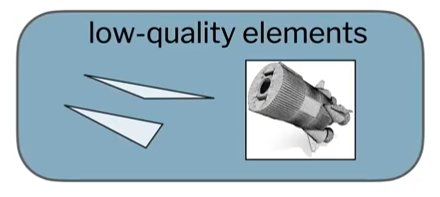These lead to high approximation error and poor numerical conditioning

Particularly relevant to this work are problems with mesh connectivity: for instance non manifold vertices or non manifold edges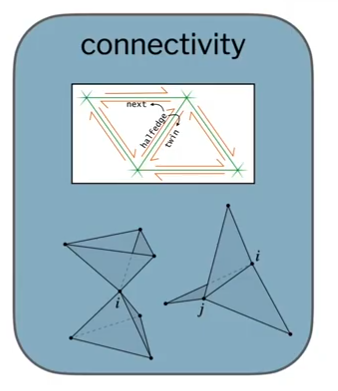These might disagree with your formulation for a problem and very pragmatically just cause your code to crash or prevent view from using certain techniques.

In particular, once we start talking about non manifold connectivity you might ask “does a non manifold Laplacian even make sense”? Because classically the laplacian is defined in local coordinates on a surface. The good news is Laplacians really are plenty meaningful on non-manifold domains.

You can see this in a lot of ways:

• one way is to think about a laplacian as a deviation from some average value
• or you can imagine just taking the variation of surface area on a non-manifold mesh
• more tangibly you could imagine taking some plates of sheet metal and welding them together in a non manifold configuration and watching how heat diffuses along this object

Along this lines we performed a simple experiment where we took this non manifold shape nd performed a harmonic interpolation problem in it using our laplacian:And then similarly slightly thickened the shape, built a tetrahedral mesh and performed in the same harmonic interpolation problem: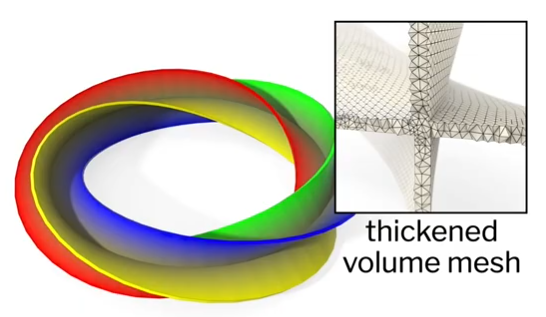You can see that the results are basically identical, which should start to give you some intuition for how this non manifold laplacian behaves.

So let's talk a little bit more precisely about how our method works. So as a reminder we're going to take us input any triangle mesh without restrictions on connectivity and output a high quality V by V laplace matrix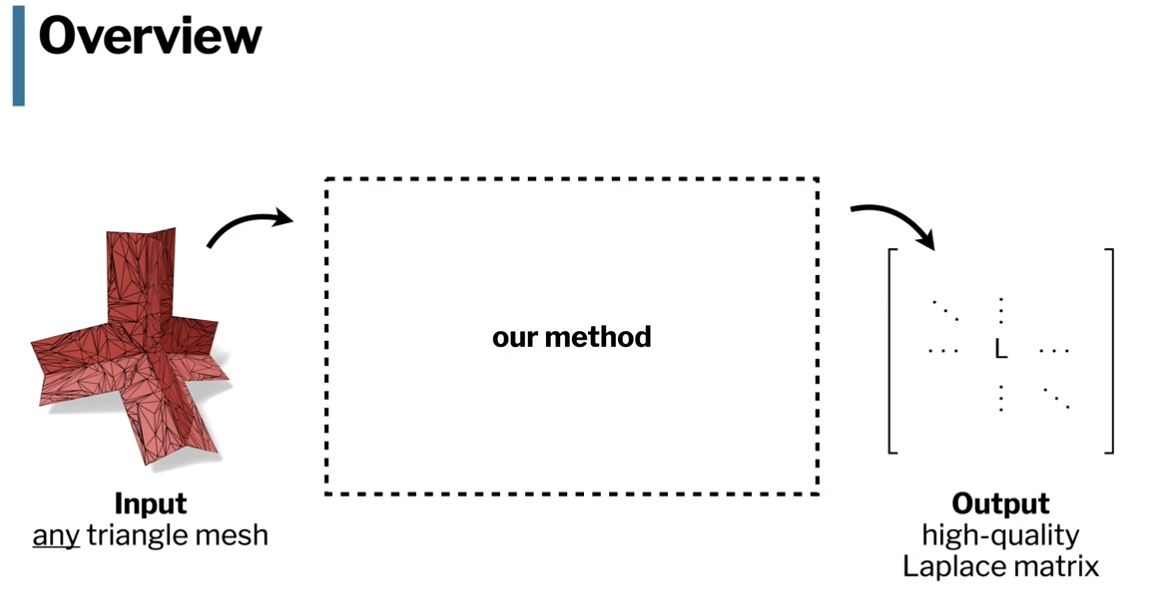And the way our method works, is that we're going to build what we call the tufted cover of this input domain and then perform intrinsic Delaunay edge flips on the tufted cover to build our laplacian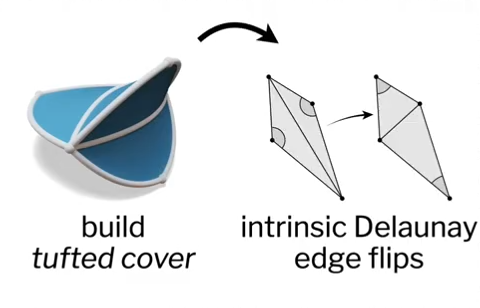This tufted cover is really the new idea because it's what allows us to handle arbitrary connectivity and do intrinsic Delaunay edge flips in the context of non manifold meshes for the first time.

An effect of this is that we're able to robustify existing algorithms. Here's a whole bunch of examples such as

harmonic interpolation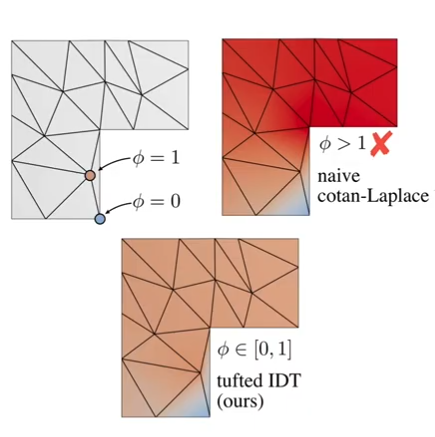differential surface editing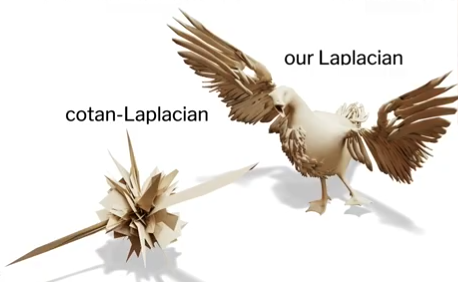and geodesic approximation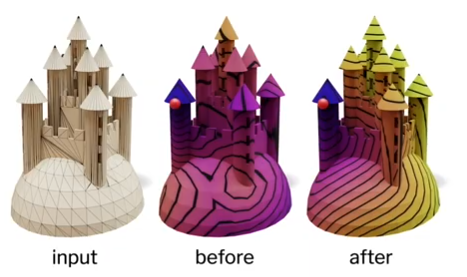and in all of these cases using the plane cotangent Laplacian gives you basically nonsensical answer, whereas just dropping in our improved Laplacian gives dramatically better results.

Some alternative strategies you might consider here would be

• Perhaps just to remesh to find some other mesh with better elements.

the downside to this is that there's no such thing as a perfect mesh, as soon as you start remeshing you have to deal with a trade-off between the number of elements, how well you approximate the underlying geometry and the quality of those elements. Ultimately it's always going to be a trade-off.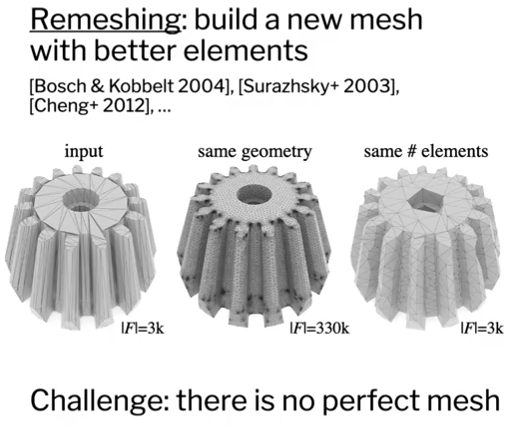• A different strategy is to go beyond linear elements and design some custom higher order basis functions.

This is really well grounded in finite element theory. Unfortunately the downside is that you generally have to go back and adapt your algorithms to use these higher-order basis functions.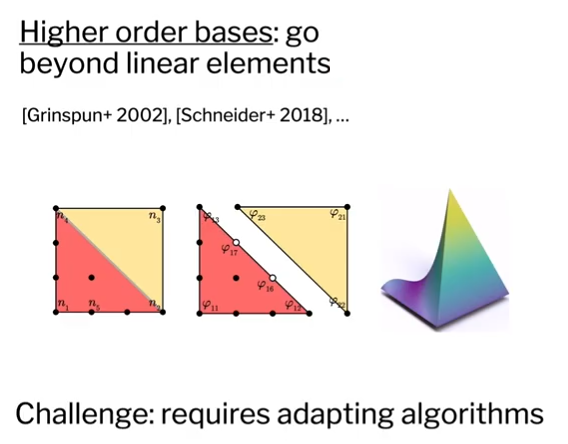I should also mention that these techniques can be dramatically more expensive than what we're proposing here. Rather taking minutes or hours you can generally build our laplacian in just a few milliseconds and it costs basically nothing more than just building the standard cotangent Laplacian.  So really what we're proposing to do is extremely efficient.

In case you're not already familiar with it, the nearly ubiquitous construction for the laplacian on triangle meshes is called the cotangent laplacian. This is a sparse symmetric matrix: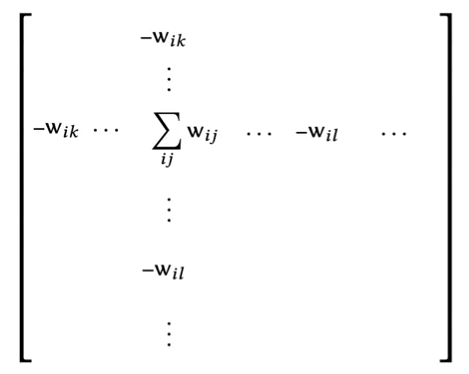Where for any pair of vertices in our mesh which are connected by some edge, we get a weight in the matrix.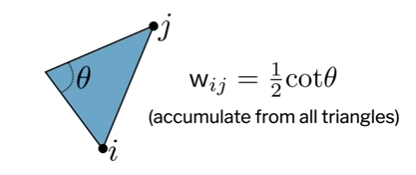That weight is given by the cotangent of the opposite angle in any triangle in which this edge appears.

You can derive this via finite elements with discrete exterior calculus (even in the context of electrical networks and so on and so forth) and I'd be remiss if I didn't mention that this is a weak laplacian so it gives integrated values which means you need a mass matrix if you want to for instance go solve a Poisson systemNow the cotangent Laplacian can already be applied to non manifold domains but there's a really big downside to the cotangent Laplacian which is that in the presence of obtuse triangles can have negative weights (that is to say that these cotangent weights are negative along some edge)This generally degrades your solution, furthermore causes your laplacian to not have the maximum principle. The maximum principle says that functions which have Lu=0 have no interior local extrema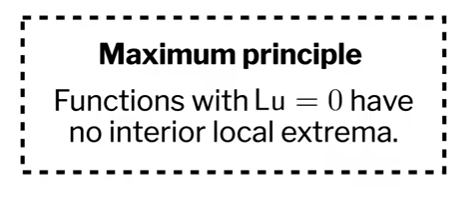Meaning, that the value at any node is going to be a convex combination of the adjacent nodes and we really want our solutions to have this maximum principle. We really want positive edge weights. Will say that if a mesh has all positive edge weights or all non-negative weights at least then it's Delaunay and meshes which are Delaunay have this maximum principle.Now the good news is that there's a fairly straightforward way to fix this and to make sure that our laplacian always has a maximum principle. But before we go into that we're going to have to take a brief aside (=make a slight digression) to talk about non manifold meshes.

So basically manifoldness just means that in a local region the mesh looks like the plane.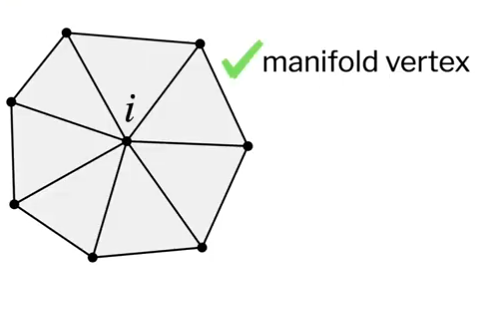You might have local regions of your mesh which don't look like the plane like for instance this non manifold vertex in an hourglass configuration: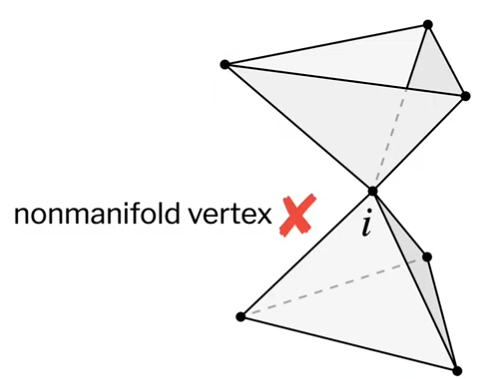or non manifold edge which has three faces incident on it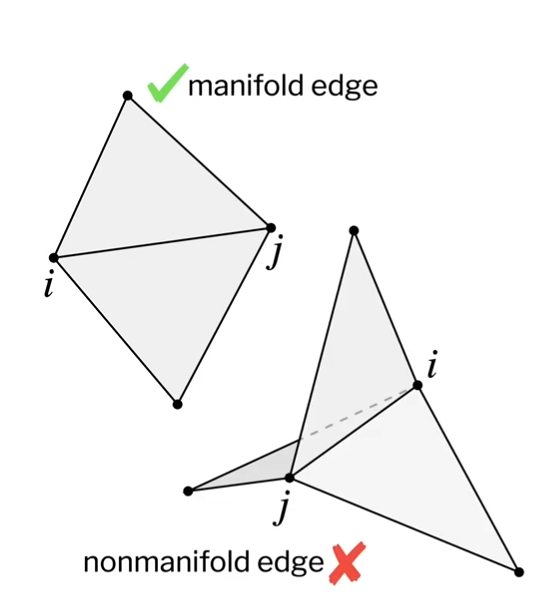sometimes non manifold meshes arise just to a tiny small feature in some larger mesh which is otherwise manifold for instance the foot of this 3d scan has just a few non manifold edges down at the bottom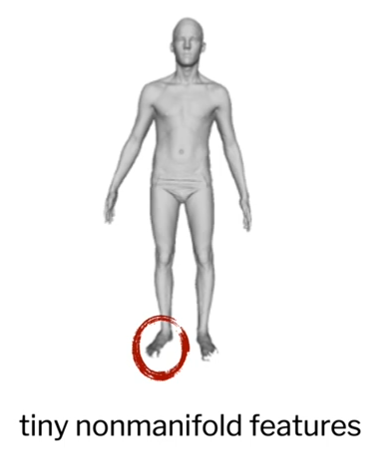or maybe more interestingly some shapes we're interested in are just outright non manifold for instance this shows up physically in in soap films when they take minimal surface configurations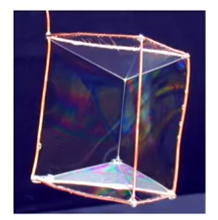and you also might see this in simulation, for instance if your simulating a fluid simulation with three interacting liquids and you extract the interface between these liquids in general it's going to be a non manifold interface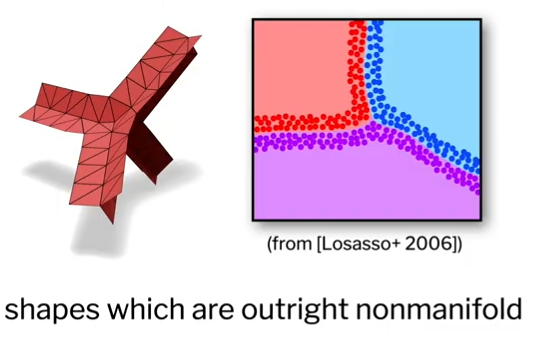so going back to the de Delaunay property and trying to get the Laplace operator with these positive edge weights. The key tool to get there is going to be intrinsic triangulations.

The basic idea of intrinsic triangulations is to forget your vertex positions and use only edge lengths.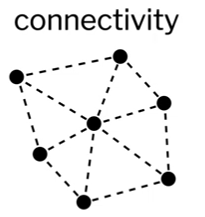So normally we might represent a mesh via its connectivity and a position associated with each vertex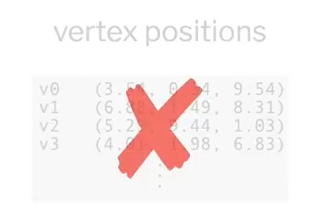but instead will represent a mesh by connectivity and a length associated with each edge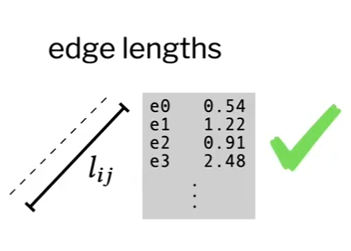this works out because you can directly evaluate many quantities directly from edge lengths. because the three side lengths of a triangle completely define its shape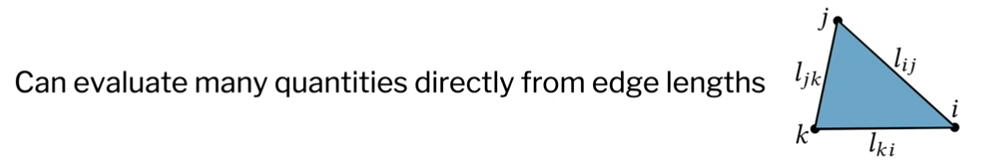Quantities which depend only on edge lengths are what we call intrinsic quantities and you guessed it the Laplacian turns out to be intrinsic.

So the really great thing about working in this intrinsic setting is that we can perform intrinsic edge flips: in particular given two triangles which are adjacent at some edge we can swap the diagonal to be the opposite diagonal within the diamond as you see here: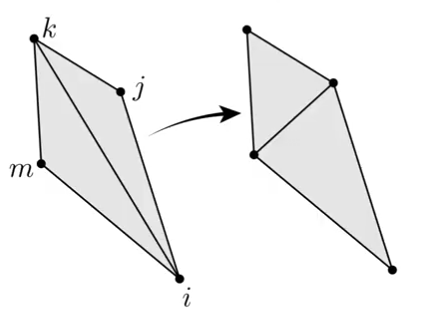And to do this computationally we just need to

• update the mesh connectivity
• and compute new edge lengths lmj

and what we're doing here, is logically constructing bent triangles that layout along the surface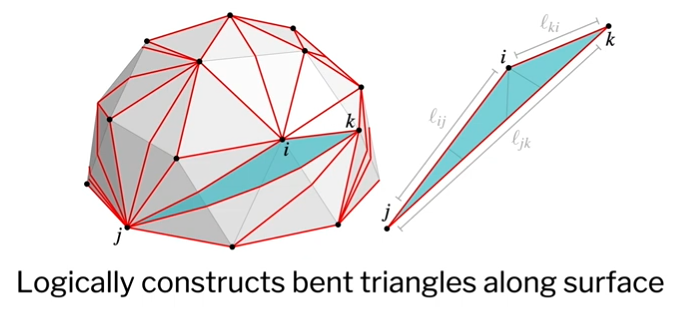Otherwise said, we're constructing a better set of basis functions for the exact same geometry. This is important because we now have the freedom to perform these edge flips and improve our basis functions thus get a better Laplace operator.

In particular the algorithm is really simple:

while any edge is not Delaunay (if it has a negative cotangent weight) you flip it!This is guaranteed to terminate with an intrinsic Delaunay mesh. here's one example on a mechanical part where the black wireframe gives the initial mesh and the colored triangles are the resulting intrinsic Delaunay triangulation: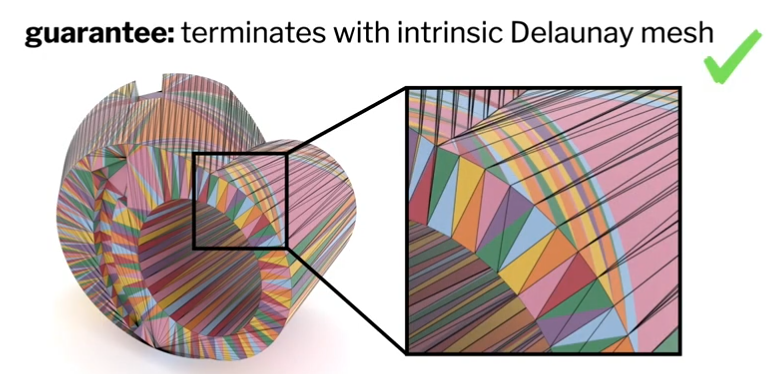Remember that our representation here is super simple: it's just ordinary mesh connectivity and a length associated with each edge (you don't have to think about the particular geometry of how these triangles sit across the surface).

So this is great and beyond giving us a positive edge weights these intrinsic Delaunay triangulations just generally improve element quality:

• We know that they maximize the minimum angle

which reduces condition number s

• and also that they discourage obtuse angles

as you see on the bottom here we get a much more natural set of basis functions on a surface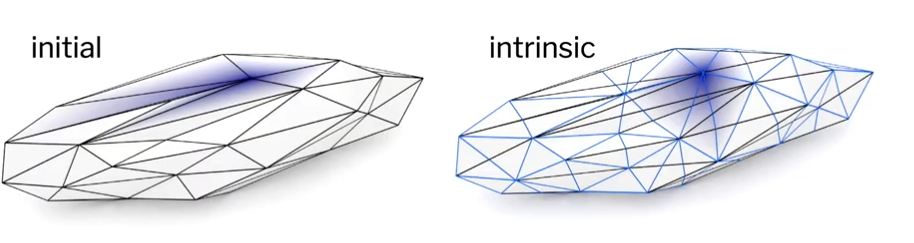Generally this also leads to a more accurate description of for instance Dirichlet energy: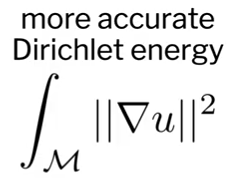so this is amazing and it's great but it has a big problem because we can't flip it on manifold edges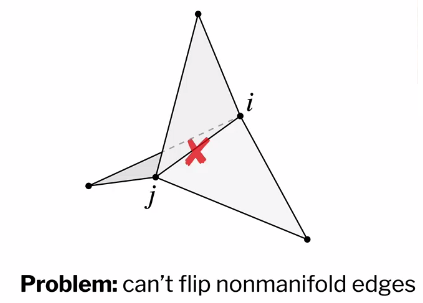and this is something that's really I think limited some of the applicability of these intrinsic Delaunay triangulations in the past because you have a very strong restriction on the connectivity.

Our big contribution is to resolve this restriction and the way we do it is really quite simple in the end, which is that we construct a particular cover of the surface.

so if our input is some impossibly non manifold triangle mesh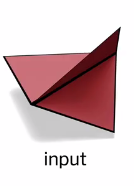we construct what we call the tufted cover of the surface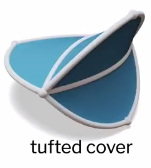and then build the intrinsic Delaunay triangulation on that tufted coverthen the laplacian of this intrinsic Delaunay triangulation of the tufted cover is our Tufted laplacian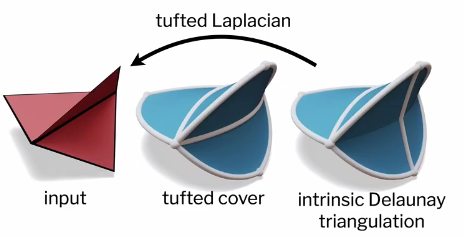and our namesake here is tufted upholstery because we feel that this cover looks a bit like the the buttons and fabric you see on top to the upholstery where the vertices are acting as the buttons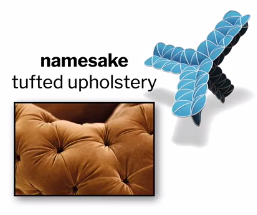one note here is that I'll draw these Tufted cover with these curved triangles but in reality we're still geometrically computing with ordinary flat triangles this is just a visualization trick.

so how did this tufted cover work? more precisely well to construct it we'll take each face in the input and create two copies of it which we logically think of as front and back copies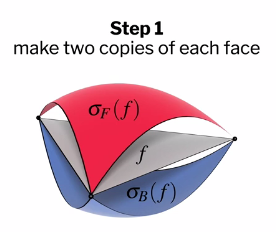and then we walk around the original edges of our mesh and glue together these front and back copies of each face along the shared edge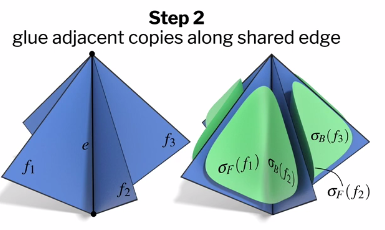so here we have front and back copies of a face and then we walk around this edge gluing front to back, front back etc at the shared side along this edge and of course we repeat this gluing procedure for every edge in the original mesh

looking at this here we have a simple non manifold mesh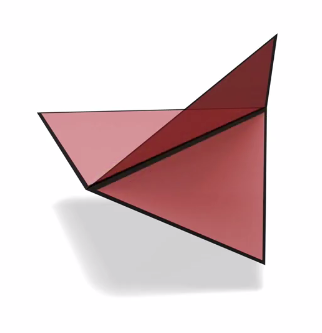which has just one non manifold edge with three faces incident on it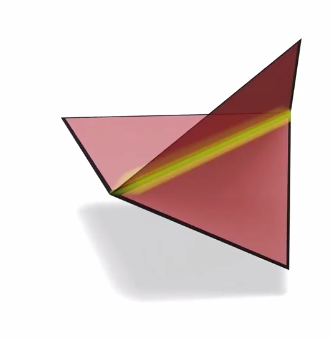to build the tufted cover we split each face into two so you get a front and back copyand then glue them together along their sides at the shared edge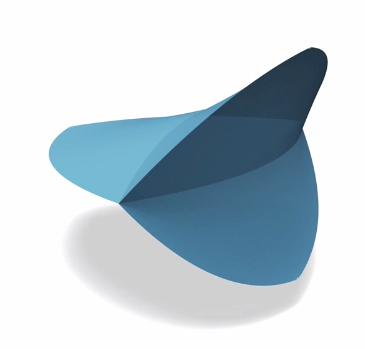this results in a new set of edges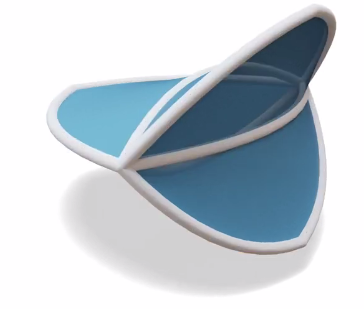and most importantly what's happened is that our non manifold edge has now become three manifold edges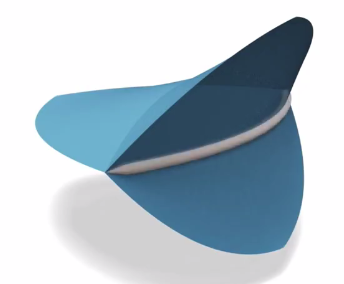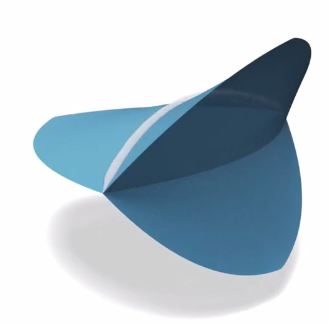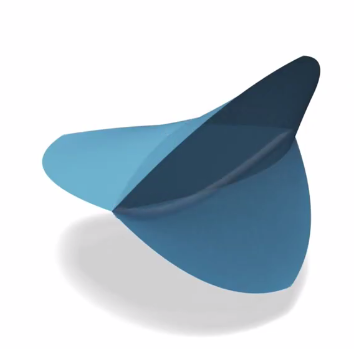Since all the edges are manifold we can perform intrinsic Delaunay edge flips and get a high-quality laplacian.

so there are some really strong guarantees that come with this tufted cover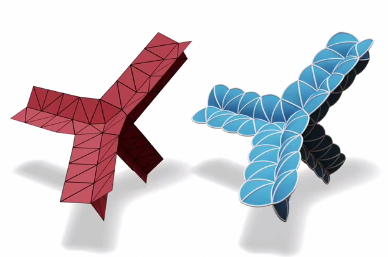in particular

- it can be constructed for any triangle mesh.

without concerns for non manifoldness or an ability or our boundary

• the tufted cover is always edge manifold
• it's always closed it's also always orientable
• In particular being closed and edge manifold means that we can always flip the edges to make it intrinsic Delaunay to make sure all these cotangent weights are positive.

we've played a funny game here, because although our construction resolved non manifold edges it actually creates non manifold vertices basically everywhere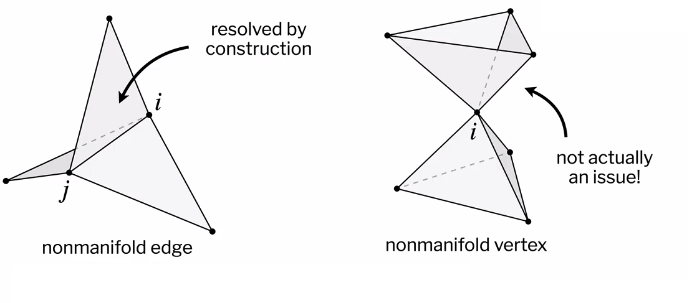but this is totally fine because non manifold vertices don't affect the Laplace operator. The outcome here is that we've generated a V by V Laplacian matrix which is a drop-in replacement for cotangent Laplace. This is really effective and allows us to immediately improve the accuracy of existing algorithms.

I mentioned that we're going to be able to handle even numerical floating-point issues as well. We do that with a very simple strategy. Numerically you can run into trouble with an intrinsic representation if your edge lengths fail to satisfy the triangle inequality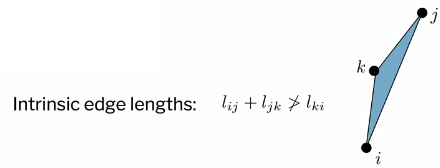and typically what will happen is your edge lengths might just barely fail to satisfy the triangle inequality in floating-point. so the simple fix is that we just add a tiny epsilon to every intrinsic edge length.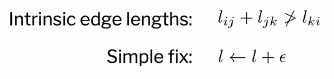In particular we will choose this epsilon to make sure that the triangle inequality is satisfied with some small buffer in every face. You might say this is so simple why are you even doing this but this is great because it's simple. If you worked with vertex positions there's no equivalent operation because if you tried to move a vertex to make one face less degenerate you might make another face more degenerate. but with the intrinsic representation we have this really simple operation “just add epsilon to your edge lengths” which gives some very pragmatic robustness to floating-point concerns.

So let's look at a few applications and experiments making use of our tufted cover. First we quite simply just validated this on some data sets. So in two large data sets which include thousands of non manifold and numerically degenerate numerically degenerate meshes, we were able to generate an intrinsic Delaunay laplacian which has a maximum principle on every single mesh.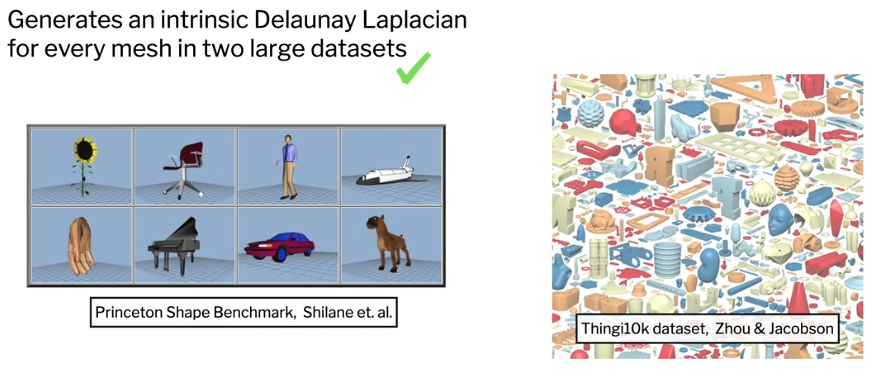This laplacian also has some really interesting consequences even just for planar domains with manifold meshes. Because we've guaranteed that all edge weights are positive in our triangulation and our Tufted triangulation it gives a very strong guarantee about bounded interpolation. In particular here if I pin down values at 0 & 1 at two vertices of the simple planar triangle mesh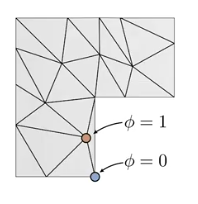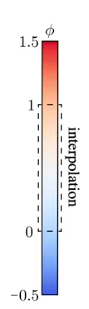and then harmonically interpolate using cotangent Laplace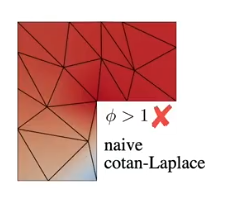we get these crazy values much greater than 1 all along the top of the mesh and the same thing happens with classic IDT (Intrinsic Delaunay Triangulation)the reason for this is that the offending edge is at the boundary and you can't flip boundary edges with normal intrinsic Delaunay triangulation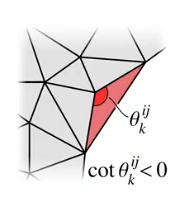However, our Tufted intrinsic Delauney triangulation can flip every edge in the mesh, thus really has all positive edge weights. Thanks to this, you get a very strong guarantee that the output of any interpolation from pinned values will always be bounded within the range of your input values.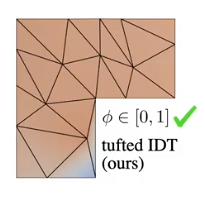We can use our a laplacian to compute minimal surfaces and here we get a lot of robustness to poor quality triangles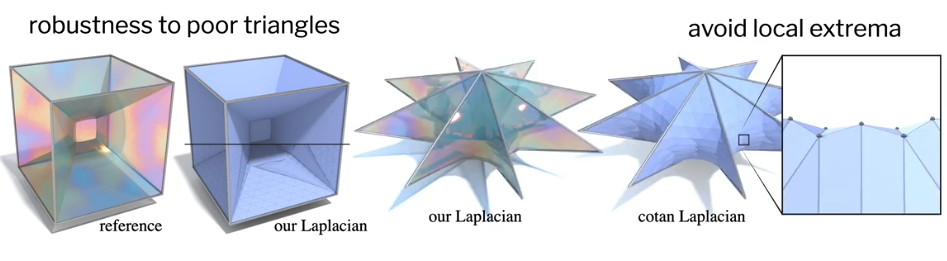and the finding minimal surfaces in terms of an intrinsic laplacian also avoids some funny artifacts you might otherwise have in the solution like local extrema.

We can use our laplacian to improve geodesic distance algorithms for instance here taking a method for computing approximate geodesic distance and running it naively on this low-quality triangle mesh yields essentially nonsense, but just dropping in our improved cotangent weights immediately greatly improves the solution.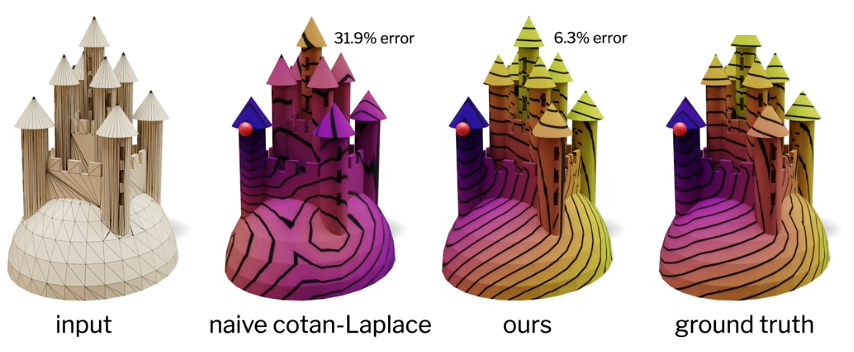we can also do this with differential surface editing where you use a numerical scheme involving a Laplacian to compute a deformation of a shape.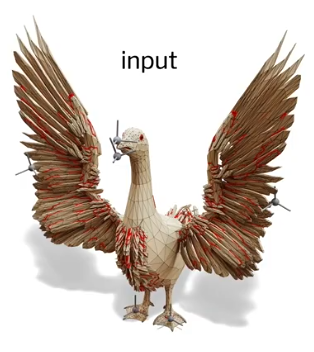Doing this naively cotangent laplace laplace yields basically numerical noise: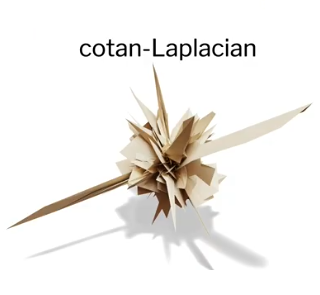but swapping in our laplacian yields a much more reasonable output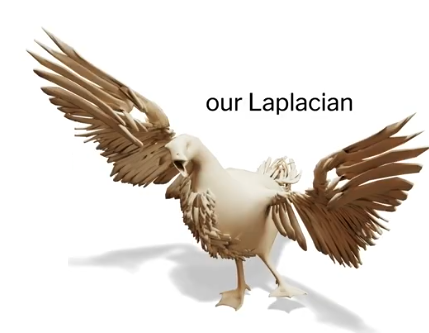And the story continues for things like spectral methods and you can do this for shape matching parameterization problems and generally across the board you'll see that using this laplacian improves the performance of existing algorithms.

So I promise that we'll also be able to build a point cloud laplacian using our method and it's going to turn out to have a lot of the same guarantees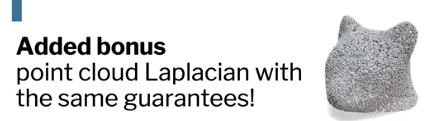in particular independently at each point we'll gather a small number of neighbors compute a normal and then project these neighbors into the tangent plane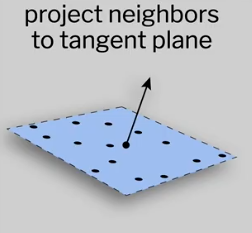We then compute a planar Delaunay triangulation in this tangent plane and adjust the triangles incident on the center vertex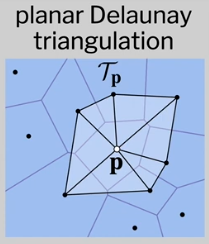taking the union of all of these local triangulations then gives us this crazy mesh which has lots of repeated faces it's non manifold all over the place and as self intersections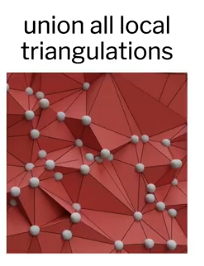but just the same we can build our tufted Laplacian and get a high-quality Laplace operator for the point cloud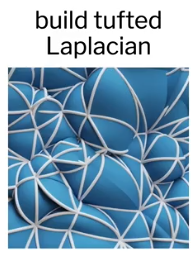this is great because there's

• no finicky numerical parameters
• and you get good sparsity properties
• and you still get a guarantee of a maximum principle

Comparing this to a few passed point clouds Laplacian. The Belkin Laplacian has great theoretical convergence properties but in practice tuning numerical parameters means that you end up getting underflow in the solution.

Here we're just computing a Green's function from a point in a scanned point cloud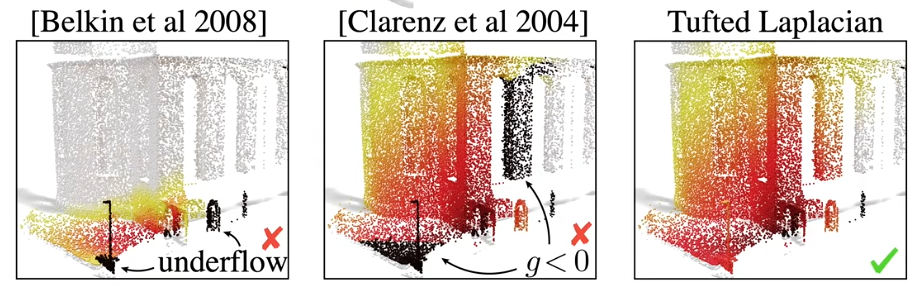computing this green's function with the laplacian of clarence et al is much better but it doesn't have a maximum principle, so you get negative values in our green's function, whereas, our Tufted laplacian gives exactly the expected solution.

You can use this also to adapt higher level algorithms to point clouds for instance here we perform spectral conformal parameterization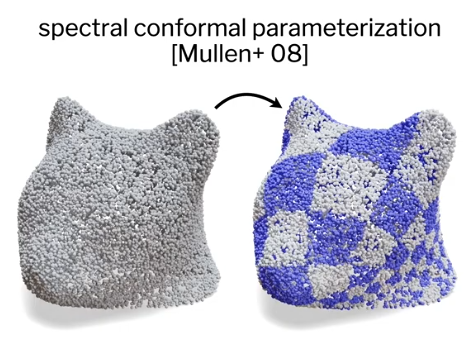as well as building a logarithmic map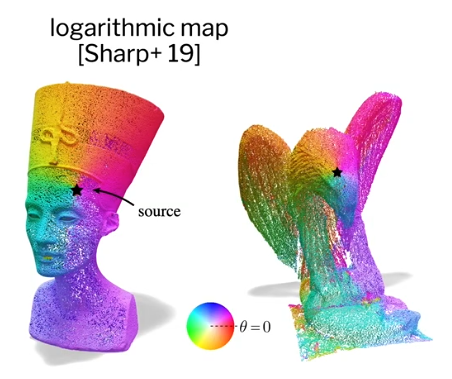Laplacian w/ maximum principle for any triangle mesh

Looking forward, our method is able to generate a high quality laplacian with a maximum principle for any triangle mesh without any restrictions on connectivity.

Drop in VxV replacement for cotan-Laplace

and it's a drop in V by V replacement for cotan Laplace

Point Cloud Laplacian

we also show how it's used to generate a point cloud laplacian

Better understanding of finite elements?

some things we'd like to continue investigating include a better understanding of the finite element implications of this construction

Build other operators on tufted cover?

as well as, whether it makes sense to build other operators beyond the laplacian on our custom cover.

Intrinsic refinement, refinement of point clouds?

and finally we'd like to investigate intrinsic refinement using this tufted cover and this might perhaps lead to a scheme for refinement of point clouds.

so if you're interested in implementing this yourself we actually describe a simple data structure in the paper which allows you to just track one additional matrix which is an edge blowing map and makes the scheme surprisingly easy to implement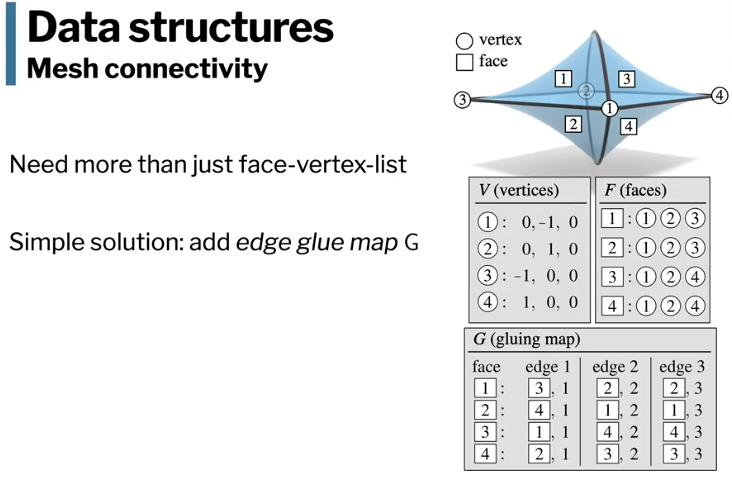but if you don't want to implement it we've already done it for you and there's a code base here which I'll link below which build the mesh and point-cloud laplacian exports the matrices in a standard format

coming soon this will be released as a Python package as well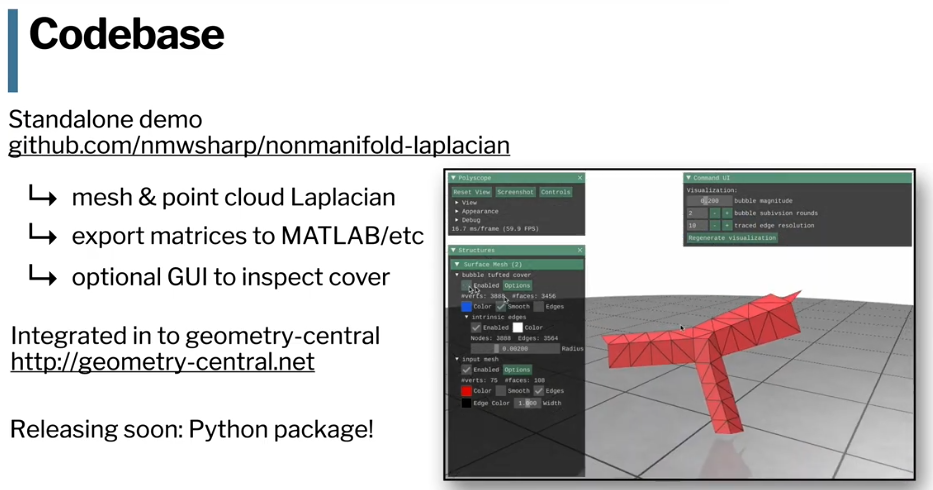https://github.com/nmwsharp/nonmanifold-laplacian

https://geometry-central.net/

so with that I'll wrap up and thank you and please don't hesitate to reach out if you have any further questions or want to chat more about this algorithm or anything else thanks